## RS Aggarwal Class 6 Solutions Chapter 5 Fractions Ex 5C

These Solutions are part of RS Aggarwal Solutions Class 6. Here we have given RS Aggarwal Solutions Class 6 Chapter 5 Fractions Ex 5C.

Other Exercises

Question 1.
Solution:
(i) $$\\ \frac { 2 }{ 3 }$$
= $$\\ \frac { 2X2 }{ 3X2 }$$
= $$\\ \frac { 4 }{ 6 }$$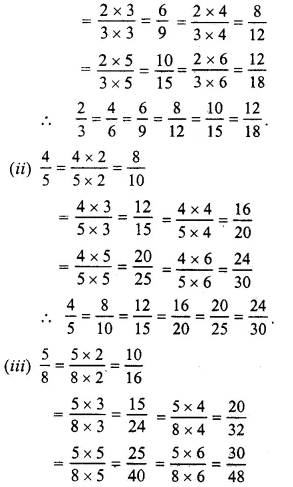Question 2.
Solution:
(i) In $$\\ \frac { 5 }{ 6 }$$ and $$\\ \frac { 20 }{ 24 }$$
$$\\ \frac { 5 }{ 6 }$$ = $$\\ \frac { 20 }{ 24 }$$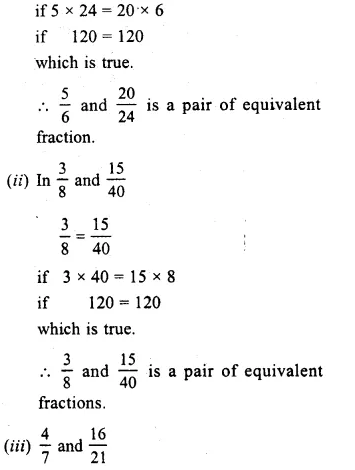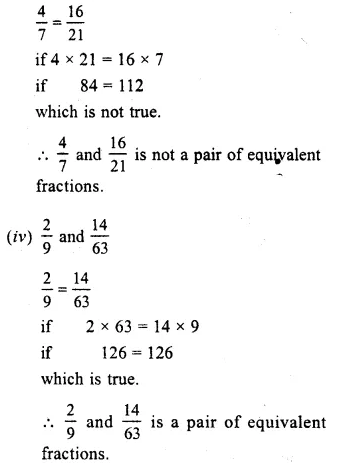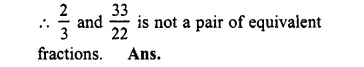Question 3.
Solution:
Equivalent fraction of $$\\ \frac { 3 }{ 5 }$$ having
(i) Denominator = 30 and 30 = 5 x 6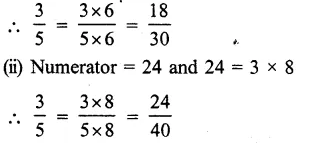Question 4.
Solution:
(i) Denominator = 54, and 54 = 9 x 6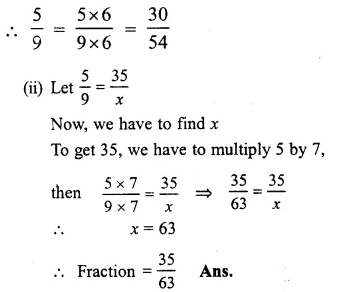Question 5.
Solution:
Equivalent fraction of $$\\ \frac { 6 }{ 11 }$$ having
(i) Denominator = 77 and 77 = 11 = 7
$$\\ \frac { 6 }{ 11 }$$
= $$\\ \frac { 6X7 }{ 11X7 }$$
= $$\\ \frac { 42 }{ 77 }$$
(ii) Numerator = 60 and 60 = 6 x 10
$$\\ \frac { 6 }{ 11 }$$
= $$\\ \frac { 6X10 }{ 11X10 }$$
= $$\\ \frac { 60 }{ 110 }$$

Question 6.
Solution:
Let $$\\ \frac { 24 }{ 30 }$$ = $$\\ \frac { 4 }{ x }$$
In order to get 4, divide 24 by 6,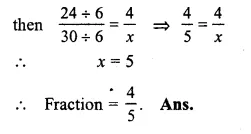Question 7.
Solution:
Equivalent fraction of $$\\ \frac { 36 }{ 48 }$$, with
(i) Numerator 9 and 9 = 36 + 4
$$\frac { 36 }{ 48 } =\frac { 36\div 4 }{ 48\div 4 } =\frac { 9 }{ 12 }$$
(ii) Denominator = 4 and 4 = 48 ÷ 12
$$\frac { 36 }{ 48 } =\frac { 36\div 12 }{ 48\div 12 } =\frac { 3 }{ 4 }$$

Question 8.
Solution:
Equivalent fraction of $$\\ \frac { 56 }{ 70 }$$ with
(i) Numerator 4 and = 56 ÷ 14
$$\frac { 56 }{ 70 } =\frac { 56\div 14 }{ 70\div 14 } =\frac { 4 }{ 5 }$$
(ii) Denominator =10 and 10 = 70 ÷ 7
$$\frac { 56 }{ 70 } =\frac { 56\div 7 }{ 70\div 7 } =\frac { 8 }{ 10 }$$

Question 9.
Solution:
(i) In $$\\ \frac { 9 }{ 15 }$$, HCF of 9 and 15 = 3
Now, dividing each term by 3, we get:
$$\frac { 9 }{ 15 } =\frac { 9\div 3 }{ 15\div 3 } =\frac { 3 }{ 5 }$$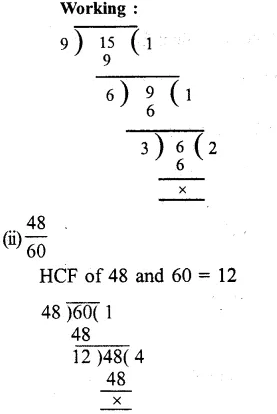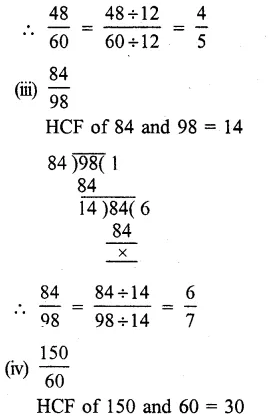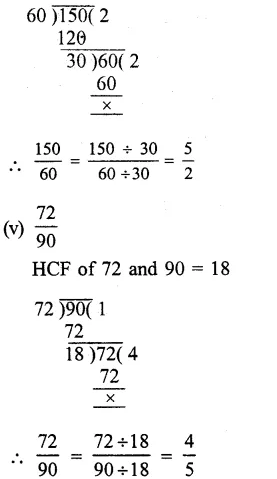Question 10.
Solution:
We know that a fraction is in its simplest form if its HCF of numerator and denominator is 1.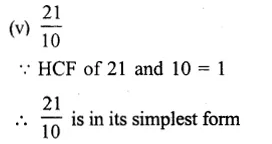Question 11.
Solution: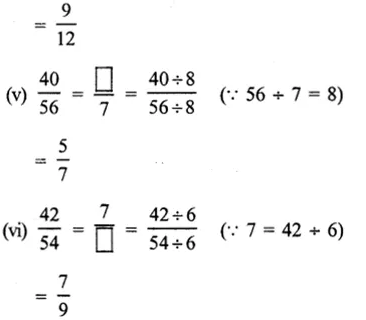Hope given RS Aggarwal Solutions Class 6 Chapter 5 Fractions Ex 5C are helpful to complete your math homework.

If you have any doubts, please comment below. Learn Insta try to provide online math tutoring for you.